StatLect

# Mean squared error of an estimator

The mean squared error (MSE) of an estimator is a measure of the expected losses generated by the estimator.

• we briefly review some concepts that are essential to understand the MSE;

• we provide a definition of MSE;

• we derive the decomposition of the MSE into bias and variance.

We will always assume, unless stated otherwise, that the parameter to be estimated is a vector.## Estimator

Letbe an unknown parameter to be estimated.

An estimator of, denoted by, is a pre-defined rule that produces an estimate offor each possible sample we can observe.

In other words,is a random variable, influenced by sampling variability, whose realizations are equal to the estimates of.

## Loss function

A loss function is a functionthat quantifies the losses generated by the estimation errors## Risk

Since the estimatoris random, we can compute the expected value of the loss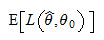which is called statistical risk.

## Definition

When a loss function called squared error is used, then the statistical risk is called mean squared error.

Definition Letbe an estimator of an unknown parameter. When the squared error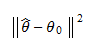is used as a loss function, then the risk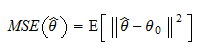is called the mean squared error of the estimator.

In this definition,is the Euclidean norm of a vector, equal to the square root of the sum of the squared entries of the vector.

## Scalar case

Whenis a scalar, the squared error is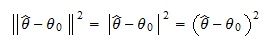because the Euclidean norm of a scalar is equal to its absolute value.

Therefore, the MSE becomes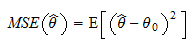## Bias variance decomposition

The following decomposition is often used to distinguish between the two main sources of error, called bias and variance.

Proposition The mean squared error of an estimatorcan be written as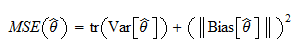whereis the trace of the covariance matrix ofand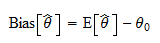is the bias of the estimator, that is, the expected difference between the estimator and the true value of the parameter.

Proof

Suppose the true parameter and its estimator are column vectors. Then, we can write: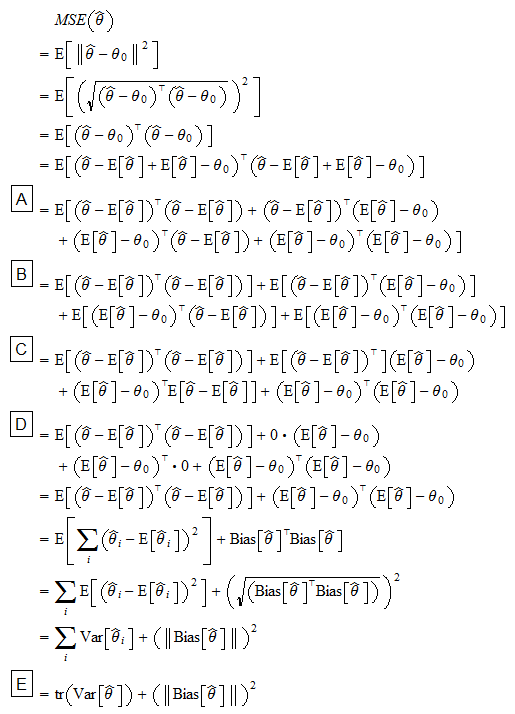where: in stepwe have expanded the products; in steps,andwe have used the linearity of the expected value operator; in stepwe have used the fact that the trace of a square matrix is equal to the sum of its diagonal elements.

When the parameteris a scalar, the above formula for the bias-variance decomposition becomes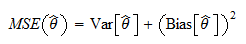Thus, the mean squared error of an unbiased estimator (an estimator that has zero bias) is equal to the variance of the estimator itself.

## More details

In the lecture on point estimation, you can find more details about:

• loss functions;

• statistical risk;

• the mean squared error.

In the lecture on predictive models, you can find a different definition of MSE that applies to predictions (not to parameter estimates).

## Keep reading the glossary

Previous entry: Mean

Next entry: Model misspecification